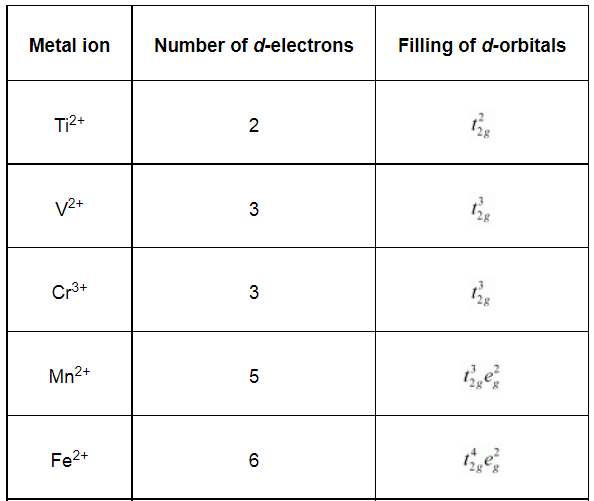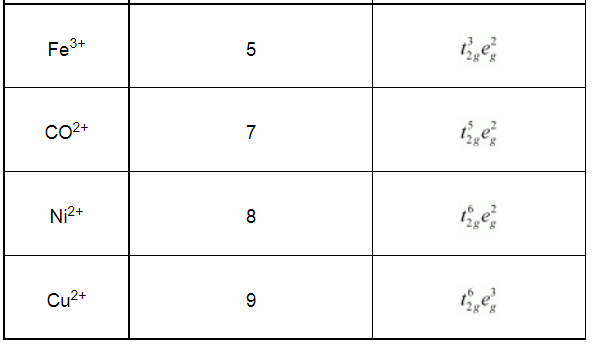# Write down the number of 3d electrons in each of the following ions:Question:

Write down the number of 3d electrons in each of the following ions:

Ti2+, V2+, Cr3+, Mn2+, Fe2+, Fe3+, CO2+, Ni2+ and Cu2+.

Indicate how would you expect the five 3d orbitals to be occupied for these hydrated ions (octahedral).

Solution: